Below is the Power Query syntax to allow for Week-on-Week comparison over multiple years.

I have seen in the past Week-on-Week comparisons but one of the challenges is what happens when it overlaps years. Especially at the start of a year like it is now Feb 2021, the users want to compare week-on-week for the past 3 months.

My challenge was to find a way to have a continuous week number over multiple years.

I knew once I had this figured out the measure would be a lot easier to compute.

## Stepping through how I got to the continuous weeks.

In the steps below I will show you how I got the final single step in Power Query.

As always, I stepped through each step, to make sure the logic worked and gave me the results I was looking for.

Put this into individual steps to show how I built the final logic.

• The first step was to calculate if this is the first year (0) or second year (1) or third year (2) based on the “Start Year” parameter
• In my parameters the start year was set to 2019
•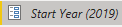• I then created the following New column to validate the data.
•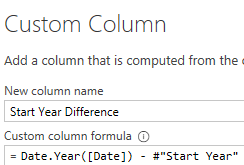• I then validated this by looking at the second year which should have 1
•• The second step was I had to get the starting point for the Week Number for the second year.
• I knew that the year 2019 had 53 weeks.
• The challenge that I had here is that I did not want the end of a year and the start of the next year to have short weeks.
• Typically, this happens because it is a new year, so the Week of Year is NOT a complete week as shown below.
•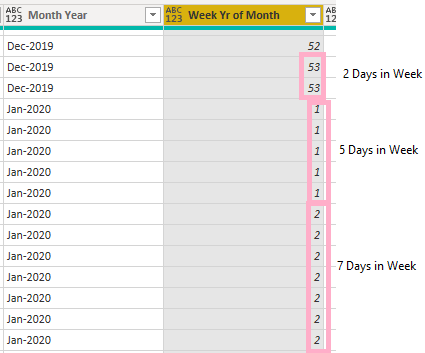• I then modified my code which would then keep a complete week for the weeks that overlap at the end of one year and the start of the next year.
•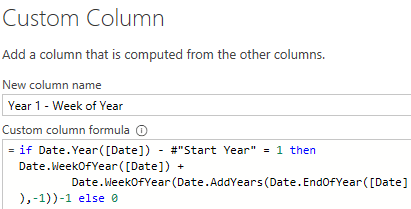• This is what it looks like when having the columns side by side to make sure it works as expected.
• I expected to see the number 53 have a total of 7 rows across the 2 columns.
•• The third step was to repeat the process, once again taking the week value from the end of the second year, keep an entire week and continue into the next year.
• This is the code that I used to get it working year 2.
•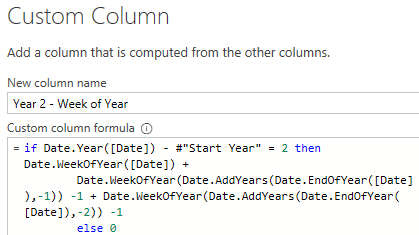• And this is what it looked like when comparing the columns.
•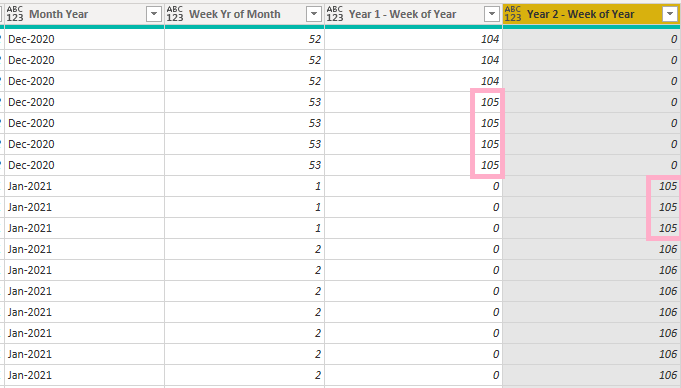• Finally, I put all of this into one step, which makes it faster and all that I want is the result.
• As shown below I get the week numbers in a continuous pattern for each year.
•• And for the next year
•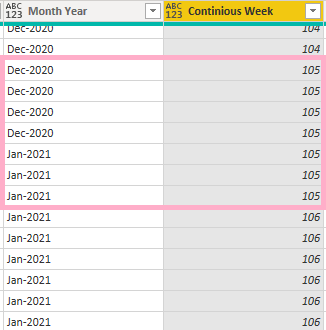• And here is the code I used to get this working below.
```#"Added Contigious Week" = Table.AddColumn(#"Changed Type29", "Contigious Week", each
// This calculates the first Year, and when it is the first year then just use the Current Week of Year
if Date.Year([Date]) - #"Start Year" = 0 then Date.WeekOfYear([Date]) + 0
// This calculates the second year, and in the second year then Get the total Number of Weeks from the Previous Year and add it to the Current Week of Year
else if Date.Year([Date]) - #"Start Year" = 1 then Date.WeekOfYear([Date]) +
Date.WeekOfYear(Date.AddYears(Date.EndOfYear([Date]),-1)) -1
// This calculates the third year, and in the third year then Add Week Number from first year, add week number from second year and add week number of current year.
else if Date.Year([Date]) - #"Start Year" = 2 then  Date.WeekOfYear([Date]) +
Date.WeekOfYear(Date.AddYears(Date.EndOfYear([Date]),-1)) -1 + Date.WeekOfYear(Date.AddYears(Date.EndOfYear([Date]),-2)) -1
else 99)
```

Here is my measure that I created for the continuous week.

```Previous Week =
CALCULATE (
SUM('Date'[Sales]),
FILTER (
ALL ( 'Date' ),

'Date'[Continious Week]
= MAX ( 'Date'[Continious Week] ) - 1
)
)
```

And this is what it looks like when shown in a table. Please note that Week 53 is the last week of the first year. It is easy now to compare proper weeks.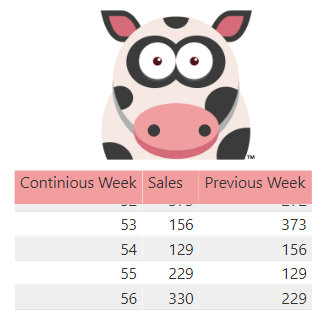## Summary

In this blog post I have shown you how to create a continuous week that keeps on going over multiple years.

This then allowed me to create a measure which compares week-on-week correctly even with overlapping years.

Thanks for reading and I hope that you found this useful!